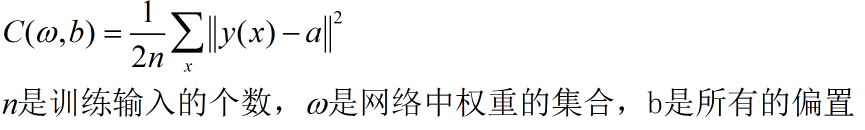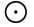第四节，Neural Networks and Deep Learning 一书小节(上)

1.使用神经网络识别手写数字2.反向传播算法如何工作

这一章作者主要介绍了反向传播的四个公式。并给出了反向传播算法的计算流程：

以MNIST数据集为例，包含50000幅用于训练的手写图片，10000幅用于校验的手写图片，10000幅用于测试的手写图片。

1.输入训练集样本的集合

2.初始化迭代期次数(epochs)，开始循环 for i in range(epochs):

2.1 打算输入训练集样本，按mini_batch_size(小批量大小)划分成许多组

2.2 针对每一小批量数据应用随机梯度下降法，并更新权重和偏置（程序中update_mini_batch(self,mini_batch,eta)函数）

2.3 一轮训练结束，用测试数据集检验准确率

3.神经网络学习结束

1.输入小批量数据的集合 mini_batch

2遍历每一个实例 (x,y)，开始循环 for x,y in mini_batch:

2.1计算每一个实例的梯度 （backprop(self,x,y)函数）

2.1.1 对每层l = 2,3,...,L（输入层记做l=1，输出层l=L），计算每一层带全权输入zl = wlal-1+bl,激活输出al = σ(zl)

2.1.2 计算输出层误差 δL=C/∂aLσ‘(zL)，计算Cx/ωLL(aL-1)T,∂Cx/∂bLL。(注意当选择不同的代价函数时δL值是不一样，

当选择二次代价函数时，δL=(aL-y)σ‘(zL)，当选择交叉熵代价函数时，δL=(aL-y))

2.1.3 反向传播误差，对每个l = L-1,L-2,...,2 计算δl = ((ωl+1)Tδl+1)σ‘(zl)，计算∂Cx/∂ωll(al-1)T,∂Cx/∂bll

2.1.4 ∂Cx/∂ω = [∂Cx/∂ω2,∂Cx/∂ω3,...,∂Cx/∂ωL]， ∂Cx/∂b = [∂Cx/∂b2,∂Cx/∂b3,...,∂Cx/∂bL]

2.2计算梯度的累积和，Σ∂Cx/∂ω，Σ∂Cx/∂b

注意：步骤2中a,b,z,y,δl均是列向量

为了提高计算的速度，步骤2我们可以采用向量化的计算方式。提示矩阵al可以写成al = [实例1al  实例2al   ....  实例mal ]

3.应用随机梯度下降法权重偏置更新法则更新权重和偏置 ω = ω-η/mΣ∂Cx/∂ω,b = b-η/mΣ∂Cx/∂b

Network1.py：非向量化方式

# -*- coding: utf-8 -*-
"""
Created on Mon Mar  5 20:24:32 2018

"""

'''

'''

import numpy as np
import random

'''

'''
def sigmod(z):
return 1.0/(1.0+np.exp(-z))

'''

'''
def sigmod_prime(z):
return sigmod(z)*(1-sigmod(z))

'''

'''
class Network(object):
'''
sizes:各层神经元的个数
weights:权重，随机初始化，服从(0,1)高斯分布 weights[i]：是一个连接着第i层和第i+1层神经元权重的numpy矩阵 i=0,1...
biases：偏置，随机初始化，服从(0,1)高斯分布 biases[i]：是第i+1层神经元偏置向量 i=0,1....
'''
def __init__(self,sizes):
#计算神经网络的层数
self.num_layers = len(sizes)
#每一层的神经元个数
self.sizes = sizes
#随机初始化权重  第i层和i+1层之间的权重向量
self.weights = [np.random.randn(y,x) for x,y in zip(sizes[:-1],sizes[1:])]
#随机初始化偏置  第i层的偏置向量  i=1...num_layers
self.biases = [np.random.randn(y,1) for y in sizes[1:]]

'''
前向反馈函数，对于网络给定一个输入向量a，返回对应的输出
'''
def  feedforward(self,a):
for b,w in zip(self.biases,self.weights):
#dot矩阵乘法  元素乘法使用*
a = sigmod(np.dot(w,a) + b)
return a

'''
随机梯度下降算法：使用小批量训练样本来计算梯度(计算随机选取的小批量数据的梯度来估计整体的梯度)
training_data:元素为(x,y)元祖的列表 (x,y)：表示训练输入以及对应的输出类别  这里的输出类别是二值化后的10*1维向量
epochs:迭代期数量 即迭代次数
mini_batch:小批量数据的大小
eta:学习率
test_data:测试数据 元素为(x,y)元祖的列表 (x,y)：表示训练输入以及对应的输出类别  这里的输出就是对应的实际数字 没有二值化
'''
def SGD(self,training_data,epochs,mini_batch_size,eta,test_data=None):
if test_data:
#计算测试集样本个数
n_test = len(test_data)
#计算训练集样本个数
n = len(training_data)
#进行迭代
for j in range(epochs):
#将训练集数据打乱，然后将它分成多个适当大小的小批量数据
random.shuffle(training_data)
mini_batches = [training_data[k:k+mini_batch_size] for k in range(0,n,mini_batch_size)]
#训练神经网络
for mini_batch in mini_batches:
self.update_mini_batch(mini_batch,eta)

#每一次迭代后 都评估一次对测试集数据进行预测的准确率
if test_data:
print('Epoch {0}:  {1}/{2}'.format(j,self.evaluate(test_data),n_test))
else:
print('Epoch {0} complete'.format(j))

'''
mini_batch：小批量数据 元素为(x,y)元祖的列表 (x,y)
eta:学习率
对每一个mini_batch应用梯度下降，更新权重和偏置
'''
def update_mini_batch(self,mini_batch,eta):
#初始化为0
nabla_b = [np.zeros(b.shape) for b in self.biases]
nabla_w = [np.zeros(w.shape) for w in self.weights]
#依次对每一个样本求梯度，并求和
for x,y in mini_batch:
#计算每一个样本代价函数的梯度（∂Cx/∂ω∂Cx/∂b）
delta_nabla_b,delta_nabla_w = self.backprop(x,y)
#梯度分量求和 Σ∂Cx/∂ω
nabla_b = [nb + dnb for nb,dnb in zip(nabla_b,delta_nabla_b)]
#梯度分量求和 Σ∂Cx/∂b
nabla_w = [nw + dnw for nw,dnw in zip(nabla_w,delta_nabla_w)]
#更新权重 w = w -  η/m*Σ∂Cx/∂ω
self.weights = [w - (eta/len(mini_batch))*nw for w,nw in zip(self.weights,nabla_w)]
#更新偏置 b = b -  η/m*Σ∂Cx/∂b
self.biases = [b - (eta/len(mini_batch))*nb for b,nb in zip(self.biases,nabla_b)]

'''
计算给定一个样本二次代价函数的梯度 单独训练样本x的二次代价函数 C = 0.5||y - aL||^2 = 0.5∑(yj - ajL)^2
返回一个元组(nabla_b,nabla_w) = ∂Cx/∂ω，∂Cx/∂b） ：和权重weights,偏置biases维数相同的numpy数组
'''
def backprop(self,x,y):
#初始化与self.baises,self.weights维数一样的两个数组 用于存放每个训练样本偏导数的累积和
nabla_b = [np.zeros(b.shape) for b in self.biases]
nabla_w = [np.zeros(w.shape) for w in self.weights]
#前向反馈
activation = x
#保存除了输入层外所有层的σ(z)的值
activations = [x]
#保存除了输入层外所有层的z的值
zs = []
#计算除了输入层外每一层z和σ(z)的值
for b,w in zip(self.biases,self.weights):
z = np.dot(w,activation) + b
zs.append(z)
activation = sigmod(z)
activations.append(activation)

#计算输出层误差
delta = self.cost_derivative(activations[-1],y)*sigmod_prime(zs[-1])
nabla_b[-1] = delta
nabla_w[-1] = np.dot(delta,activations[-2].transpose())

#计算反向传播误差
for l in range(2,self.num_layers): z = zs[-l] sp = sigmod_prime(z) delta = np.dot(self.weights[-l+1].transpose(),delta)*sp nabla_b[-l] = delta nabla_w[-l] = np.dot(delta,activations[-l-1].transpose()) return (nabla_b,nabla_w) ''' 对神经网络预测准确率进行评估 ''' def evaluate(self,test_data): #np.argmax返回最大值所在的索引 这里获取预测数值和实际数值组成元组的列表 test_results = [(np.argmax(self.feedforward(x)),y) for x,y in test_data] #计算预测值 == 实际值的总个数 return sum(int(x==y) for x,y in test_results) ''' 计算损失函数的偏导数C/a a是实际输出 ''' def cost_derivative(self,output_activations,y): return (output_activations - y)

def  network_baseline():
#遇到编码错误：参考链接http://blog.csdn.net/qq_41185868/article/details/79039604S
#traning_data:[(784*1,10*1),...]，50000个元素
#validation_data[(784*1,1*1),....],10000个元素
#test_data[(784*1,1*1),....],10000个元素
print('训练集数据长度',len(training_data))
print(training_data.shape)      #训练集每一个样本的特征维数   （784，1）
print(training_data.shape)      #训练集每一个样本对应的输出维数  （10，1）

print('测试集数据长度',len(test_data))
print(test_data.shape)         #测试机每一个样本的特征维数,1,1   （784,1）
#print(test_data.shape)         #测试机每一个样本对应的输出维数   （） 这里与训练集的输出略有不同，这里输出是一个数 并不是二指化后的10*1维向量
print(test_data)               #7

#测试
net = Network([784,30,10])
'''
print(net.num_layers)      #3
print(net.sizes)
print(net.weights)
print(net.biases)
'''

net.SGD(training_data,30,10,3.0,test_data=test_data)

#运行程序
network_baseline()

向量化方式：

# -*- coding: utf-8 -*-
"""
Created on Mon Mar  5 20:24:32 2018

"""

'''

'''

import numpy as np
import random

'''

'''
def sigmod(z):
return 1.0/(1.0+np.exp(-z))

'''

'''
def sigmod_prime(z):
return sigmod(z)*(1-sigmod(z))

'''

'''
class Network(object):
'''
sizes:各层神经元的个数
weights:权重，随机初始化，服从(0,1)高斯分布 weights[i]：是一个连接着第i层和第i+1层神经元权重的numpy矩阵 i=0,1...
biases：偏置，随机初始化，服从(0,1)高斯分布 biases[i]：是第i+1层神经元偏置向量 i=0,1....
'''
def __init__(self,sizes):
#计算神经网络的层数
self.num_layers = len(sizes)
#每一层的神经元个数
self.sizes = sizes
#随机初始化权重  第i层和i+1层之间的权重向量
self.weights = [np.random.randn(y,x) for x,y in zip(sizes[:-1],sizes[1:])]
#随机初始化偏置  第i层的偏置向量  i=1...num_layers
self.biases = [np.random.randn(y,1) for y in sizes[1:]]

'''
前向反馈函数，对于网络给定一个输入向量a，返回对应的输出
'''
def  feedforward(self,a):
for b,w in zip(self.biases,self.weights):
#dot矩阵乘法  元素乘法使用*
a = sigmod(np.dot(w,a) + b)
return a

'''
随机梯度下降算法：使用小批量训练样本来计算梯度(计算随机选取的小批量数据的梯度来估计整体的梯度)
training_data:元素为(x,y)元祖的列表 (x,y)：表示训练输入以及对应的输出类别  这里的输出类别是二值化后的10*1维向量
epochs:迭代期数量 即迭代次数
mini_batch:小批量数据的大小
eta:学习率
test_data:测试数据 元素为(x,y)元祖的列表 (x,y)：表示训练输入以及对应的输出类别  这里的输出就是对应的实际数字 没有二值化
'''
def SGD(self,training_data,epochs,mini_batch_size,eta,test_data=None):
if test_data:
#计算测试集样本个数
n_test = len(test_data)
#计算训练集样本个数
n = len(training_data)
#进行迭代
for j in range(epochs):
#将训练集数据打乱，然后将它分成多个适当大小的小批量数据
random.shuffle(training_data)
mini_batches = [training_data[k:k+mini_batch_size] for k in range(0,n,mini_batch_size)]
#训练神经网络
for mini_batch in mini_batches:
self.update_mini_batch(mini_batch,eta)

#每一次迭代后 都评估一次对测试集数据进行预测的准确率
if test_data:
print('Epoch {0}:  {1}/{2}'.format(j,self.evaluate(test_data),n_test))
else:
print('Epoch {0} complete'.format(j))

'''
mini_batch：小批量数据 元素为(x,y)元祖的列表 (x,y)
eta:学习率
对每一个mini_batch应用梯度下降，更新权重和偏置  采用向量化方式计算
'''
def update_mini_batch(self,mini_batch,eta):
#初始化与self.baises,self.weights维数一样的两个数组 用于存放每个训练样本偏导数的累积和
nabla_b = [np.zeros(b.shape) for b in self.biases]
nabla_w = [np.zeros(w.shape) for w in self.weights]

#实例数
m = len(mini_batch)
#print('小批量数目',m)

#把输入样本形式转换为 [样本1列向量 样本2列向量 ...  样本m列向量]的形式
#[样本1类别列向量 样本2类别列向量 ...  样本m类别列向量]的形式
training_x = [x for x,y in mini_batch]         #(10,(784,1))
training_y = [y for x,y in mini_batch]         #(10,(10,1))

#注意这里需要转置
X = np.array(training_x).reshape(m,training_x.shape).T
Y = np.array(training_y).reshape(m,training_y.shape).T

#print('X',X.shape)                      #(784,10)
#print('Y',Y.shape)                      #(10,10)

#计算前向输出
activation = X
#保存除了输入层外所有层的σ(z)的值
activations = [X]
#保存除了输入层外所有层的z的值
zs = []
#计算除了输入层外每一层z和σ(z)的值
for b,w in zip(self.biases,self.weights):
z = np.dot(w,activation) + b
zs.append(z)
activation = sigmod(z)
activations.append(activation)

#计算输出层误差
delta = self.cost_derivative(activations[-1],Y)*sigmod_prime(zs[-1])
nabla_w[-1] = np.dot(delta,activations[-2].transpose())
#按照行求和
nabla_b[-1] = np.sum(delta,axis = 1, keepdims = True)

#计算反向传播误差
for l in range(2,self.num_layers):
delta = np.dot(self.weights[-l+1].transpose(),delta)*sigmod_prime(zs[-l])
nabla_w[-l] = np.dot(delta,activations[-l-1].transpose())
nabla_b[-l] = np.sum(delta,axis =1,keepdims=True)

#更新权重 w = w -  η/m*Σ∂Cx/∂ω
self.weights = [w - eta/m*nw for w,nw in zip(self.weights,nabla_w)]
#更新偏置 b = b -  η/m*Σ∂Cx/∂b
self.biases = [b - eta/m*nb for b,nb in zip(self.biases,nabla_b)]

'''
对神经网络预测准确率进行评估
'''
def evaluate(self,test_data):
#np.argmax返回最大值所在的索引  这里获取预测数值和实际数值组成元组的列表
test_results = [(np.argmax(self.feedforward(x)),y) for x,y in test_data]
#计算预测值 == 实际值的总个数
return sum(int(x==y) for x,y in test_results)

'''
计算损失函数的偏导数∂C/∂a  a是实际输出
'''
def cost_derivative(self,output_activations,y):
return (output_activations - y)

def  network_baseline():
#遇到编码错误：参考链接http://blog.csdn.net/qq_41185868/article/details/79039604S
#traning_data:[(784*1,10*1),...]，50000个元素
#validation_data[(784*1,1*1),....],10000个元素
#test_data[(784*1,1*1),....],10000个元素
print('训练集数据长度',len(training_data))
print(training_data.shape)      #训练集每一个样本的特征维数   （784，1）
print(training_data.shape)      #训练集每一个样本对应的输出维数  （10，1）

print('测试集数据长度',len(test_data))
print(test_data.shape)         #测试机每一个样本的特征维数,1,1   （784,1）
#print(test_data.shape)         #测试机每一个样本对应的输出维数   （） 这里与训练集的输出略有不同，这里输出是一个数 并不是二指化后的10*1维向量
print(test_data)               #7

#测试
net = Network([784,30,10])
'''
print(net.num_layers)      #3
print(net.sizes)
print(net.weights)
print(net.biases)
'''

net.SGD(training_data,30,10,3,test_data=test_data)

network_baseline()

参考文章

深度神经网络（DNN）模型与前向传播算法

深度神经网络（DNN）反向传播算法(BP)

posted @ 2018-03-24 15:29  大奥特曼打小怪兽  阅读(1304)  评论(0编辑  收藏  举报